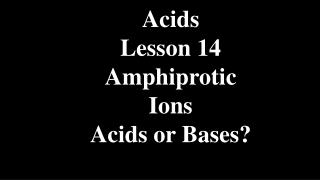DownloadDownload PresentationAcids Lesson 14 Amphiprotic Ions Acids or Bases?

Acids Lesson 14 Amphiprotic Ions Acids or Bases?

Télécharger la présentationAcids Lesson 14 Amphiprotic Ions Acids or Bases?

- - - - - - - - - - - - - - - - - - - - - - - - - - - E N D - - - - - - - - - - - - - - - - - - - - - - - - - - -
Presentation Transcript

1. Acids Lesson 14 Amphiprotic Ions Acids or Bases?

2. Amphiprotic Ions H3PO4Acid H2PO4-Amphiprotic HPO42-Amphiprotic PO43-Base Amphiprotic ionshave a Ka and a Kb. If the Ka > Kb, then the ion is an acid. If the Kb > Ka, then the ion is a base.

3. 1. Is H2PO4- an acid or base when in water? Write the predominate hydrolysis equation for this ion. Ka (H2PO4-) = 6.2 x 10-8 Kb (H2PO4-)=Kw Ka(H3PO4) =1 x 10-14 7.5 x 10-3 =1.3 x 10-12 Ka > Kb Acid H2PO4- + H2O ⇄HPO42- + H3O+

4. 2. Is HPO42- an acid or base when in water? Write the hydrolysis equation for this ion. Ka (HPO42-) = 2.2 x 10-13 Kb (HPO42-)= Kw Ka(H2PO4-) =1 x 10-14 6.2 x 10-8 =1.6 x 10-7 Kb > Ka base HPO42- + H2O ⇄H2PO4- + OH-

5. 3. Show by calculation if NH4F is an acid or base when in water. NH4F → NH4+ + F- acid base Both have weak parents- neither will cross off! What will the salt be? Ka(NH4+) = 5.6 x 10-10 Kb (F-) = = Kw Ka(HF) = 1 x 10-14 3.5 x 10-4 =2.9 x 10-11 Ka > Kbacid

6. 4. Show by calculation if NH4CH3COO is an acid or base when in water. NH4CH3COO → NH4+ + CH3COO- acid base Ka(NH4+) = 5.6 x 10-10 Kb (CH3COO-) = = Kw Ka(CH3COOH) = 1 x 10-14 1.8 x 10-5 =5.6 x 10-10 Ka = Kbneutral pH = 7.00

7. 5. Calculate the volume of 0.100 M H2CO3 required to neutralize 25.0 mL of 0.200 M KOH. 1H2CO3 +2KOH → K2CO3 + 2HOH ? L 0.0250 L 0.100 M 0.200 M

8. 5. Calculate the volume of 0.100 M H2CO3 required to neutralize 25.0 mL of 0.200 M KOH. 1H2CO3 +2KOH → K2CO3 + 2HOH ? L 0.0250 L 0.100 M 0.200 M 0.0250 L KOH

9. 5. Calculate the volume of 0.100 M H2CO3 required to neutralize 25.0 mL of 0.200 M KOH. 1H2CO3 +2KOH → K2CO3 + 2HOH ? L 0.0250 L 0.100 M 0.200 M 0.0250 L KOH x 0.200 mol L

10. 5. Calculate the volume of 0.100 M H2CO3 required to neutralize 25.0 mL of 0.200 M KOH. 1H2CO3 +2KOH → K2CO3 + 2HOH ? L 0.0250 L 0.100 M 0.200 M 0.0250 L KOH x 0.200 mol x 1 mole H2CO3 L 2 mole KOH

11. 5. Calculate the volume of 0.100 M H2CO3 required to neutralize 25.0 mL of 0.200 M KOH. 1H2CO3 +2KOH → K2CO3 + 2HOH ? L 0.0250 L 0.100 M 0.200 M 0.0250 L KOH x 0.200 mol x 1 mole H2CO3 x 1 L L 2 mole KOH 0.100 mol

12. 5. Calculate the volume of 0.100 M H2CO3 required to neutralize 25.0 mL of 0.200 M KOH. 1H2CO3+2KOH → K2CO3 + 2HOH ? L 0.0250 L 0.100 M 0.200 M 0.0250 L KOH x 0.200 mol x 1 mole H2CO3 x 1 L= 0.0250 L L 2 mole KOH 0.100 mol

13. 6. Label the equation that has a Ka, Kb, Ksp, Kw, or Keq. Mg(OH)2(s)⇌ Mg2+ + 2OH- CN- + H2O ⇌ HCN + OH- NH4+ + H2O ⇌ H3O+ + NH3   N2O4(g)⇌ 2NO2(g) 2H2O ⇌ H3O+ + OH-

14. 6. Label the equation that has a Ka, Kb, Ksp, Kw, or Keq. Ksp Mg(OH)2(s)⇌ Mg2+ + 2OH- CN- + H2O ⇌ HCN + OH- NH4+ + H2O ⇌ H3O+ + NH3   N2O4(g)⇌ 2NO2(g) 2H2O ⇌ H3O+ + OH-

15. 6. Label the equation that has a Ka, Kb, Ksp, Kw, or Keq. Ksp Mg(OH)2(s)⇌ Mg2+ + 2OH- Kb CN- + H2O ⇌ HCN + OH- NH4+ + H2O ⇌ H3O+ + NH3   N2O4(g)⇌ 2NO2(g) 2H2O ⇌ H3O+ + OH-

16. 6. Label the equation that has a Ka, Kb, Ksp, Kw, or Keq. Ksp Mg(OH)2(s)⇌ Mg2+ + 2OH- Kb CN- + H2O ⇌ HCN + OH- Ka NH4+ + H2O ⇌ H3O+ + NH3   N2O4(g)⇌ 2NO2(g) 2H2O ⇌ H3O+ + OH-

17. 6. Label the equation that has a Ka, Kb, Ksp, Kw, or Keq. Ksp Mg(OH)2(s)⇌ Mg2+ + 2OH- Kb CN- + H2O ⇌ HCN + OH- Ka NH4+ + H2O ⇌ H3O+ + NH3 Keq N2O4(g)⇌ 2NO2(g) 2H2O ⇌ H3O+ + OH-

18. 6. Label the equation that has a Ka, Kb, Ksp, Kw, or Keq. Ksp Mg(OH)2(s)⇌ Mg2+ + 2OH- Kb CN- + H2O ⇌ HCN + OH- Ka NH4+ + H2O ⇌ H3O+ + NH3 Keq N2O4(g)⇌ 2NO2(g) Kw 2H2O ⇌ H3O+ + OH-